## Samacheer Kalvi 3rd Standard Maths Book Solutions Term 1 Chapter 3 Patterns

Practice (Text Book Page No. 36):

Question 1.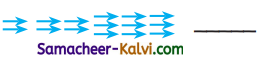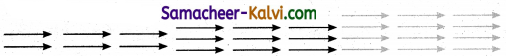Question 2.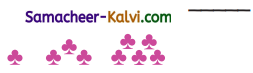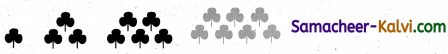Question 3.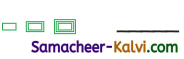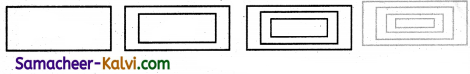Question 4.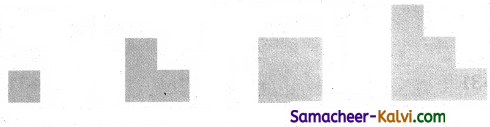b. Continue the growing pattern.

Question 1.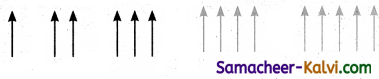Question 2.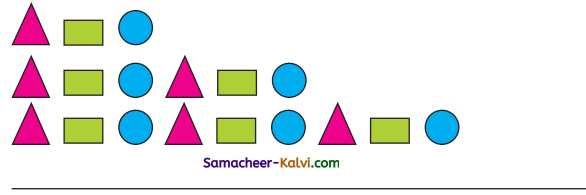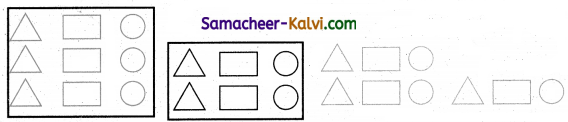Question 3.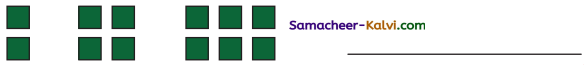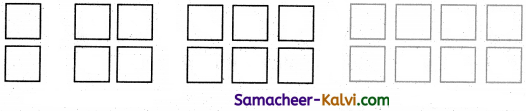Practice (Text Book Page No. 37):

Continue the repeating patterns upto 3 steps in the space Provided.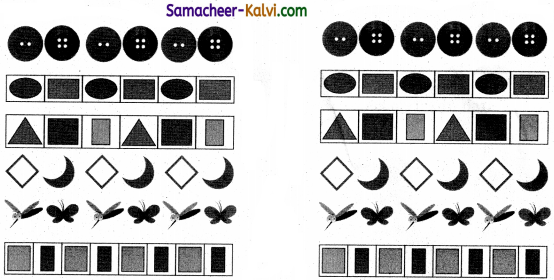Activity 3 (Text Book Page No. 37):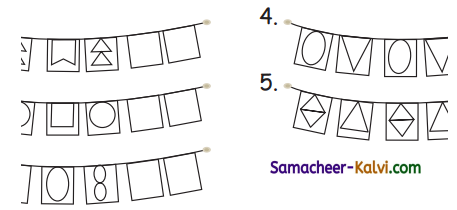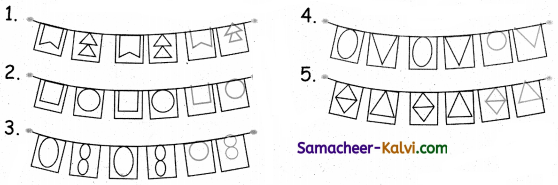Activity 4 (Text Book Page No. 38):

Question 1.
Match the following and complete the pattern.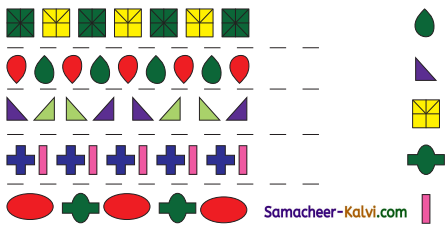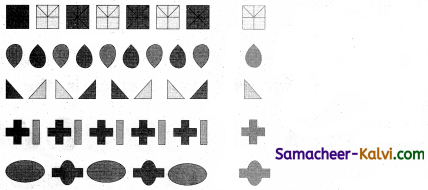Question 2.
Match the following.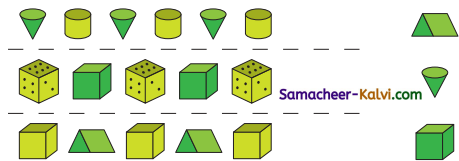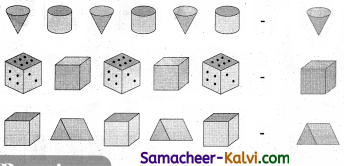Practice (Text Book Page No. 39):

Continue the Straight – line patterns.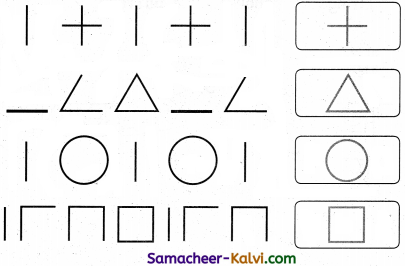Activity 5 (Text Book Page No. 42):

Complete the symmetrical image.

Question 1.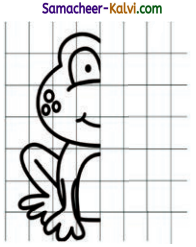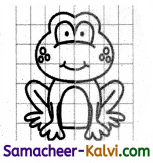Question 2.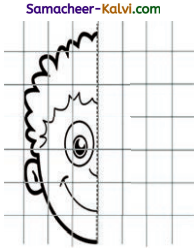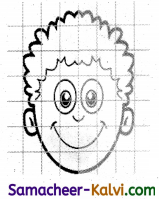Activity 6 (Text Book Page No. 43):

a. Draw the lines of symmetry for the following figures.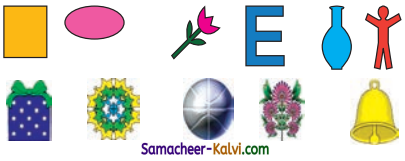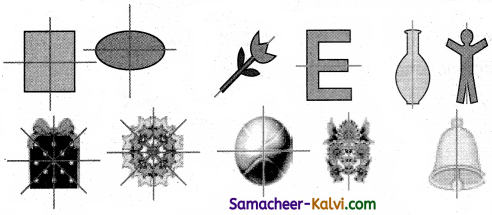b. Circle the non – symmetrical shapes.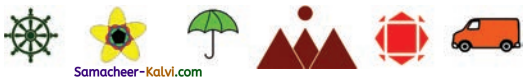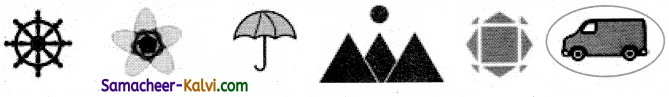Activity 7 (Text Book Page No. 43):

Draw the other half from the line of symmetry to make it symmetrical.

Question 1.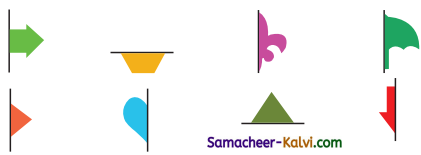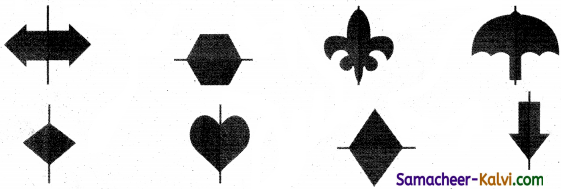Activity 8 (Text Book Page No. 43):

Line symmetry is also found in some letters of the alphabet. Complete Write the letters with line symmetry.